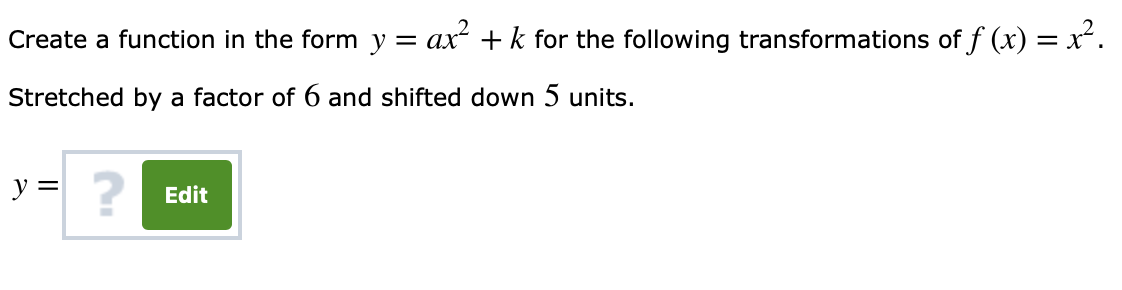# of f(x)2Create a function in the form y - ar2 +k for the followingStretched by a factor of 6 and shifted down 5 units.2Edit

Question
3 viewshelp_outlineImage Transcriptioncloseof f(x)2 Create a function in the form y - ar2 +k for the following Stretched by a factor of 6 and shifted down 5 units. 2 Edit fullscreen
check_circle

Step 1

When a function f (x) is stretched by a factor c, the transformed function is given by cf (x).

Hence, when the function f (x) = x2 is stretched by a factor of 6, the transformed function is given by F(x) = 6x2

When a function f (x) is shifted down by c units, the transformed function is given by f (x)-c.

Hence, when the function F(x) = 6x2 is ...

### Want to see the full answer?

See Solution

#### Want to see this answer and more?

Solutions are written by subject experts who are available 24/7. Questions are typically answered within 1 hour.*

See Solution
*Response times may vary by subject and question.
Tagged in

### Algebra